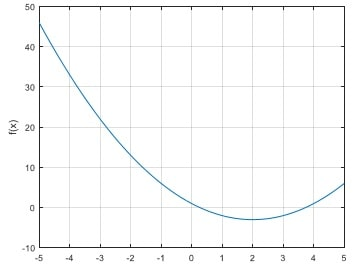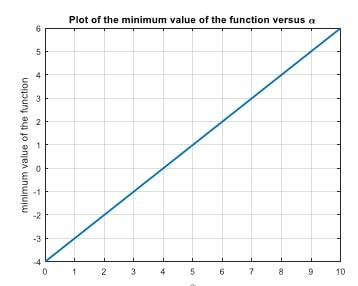# ME2500 Project 1 Example on Parametric Studies

Understanding the dependence of functions (measured variables) on system parameters is important in engineering. It forms the foundation for an engineering practice called the parametric study. Consider the following function f(x) which also depends on a parameter α

f(x) = x2 - 4x + α

For the example of α = 1, the following plot shows that there is a minimum value.

{
>> alpha = 1;
>> figure, fplot(@(x) x.^2-4*x+alpha, [-5,5]), grid
>> xlabel('x'), ylabel('f(x)')
}It can be seen that the function has a minimum at x = 2 (in this problem, for all values of α). The value of this minimum depends on the value of α. Denote this minimal value as funmin. (Avoid using the variable fmin which is the name a MATLAB built-in function.) Produce a plot of the minimal value of the function against the parameter α varying from 0 to 10. We will use the same approach outlined in the solution for part (b) of Project 1.

alpha_min = 0;    % lower bound value of alpha
alpha_max = 10;   % upper bound value of alpha
% Define the function of two variables, x and alpha
fun2=@(x,alpha) x.^2-4*x+alpha;
alpha_data = linspace(alpha_min,alpha_max);
n_alpha_data = length(alpha_data);
funmin_data = zeros(n_alpha_data,1); % initialize the vector funmin_data
for k=1:n_alpha_data
funmin_data(k) = fun2(2,alpha_data(k));
end
figure, plot(alpha_data,funmin_data,'Linewidth',2), grid
xlabel('\alpha'), ylabel('minimum value of the function')
title('Plot of the minimum value of the function versus \alpha')The above plot is consistent with the analytical result which can be shown to be

fmin = α-4

The above-outlined programming structure is useful for conducting parametric studies.